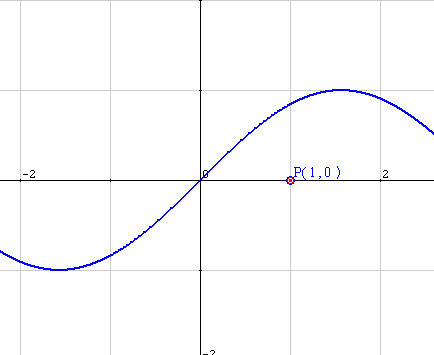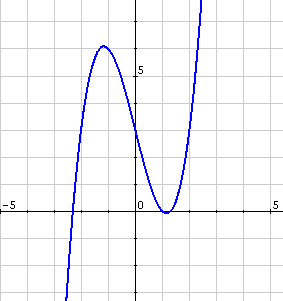# Maximun, minimum and inflection points of a function

The analysis of the functions contains the computation of its maxima, minima and inflection points (we will call them the relative maxima and minima or more generally the relative extrema). What do we mean by that?We can clearly see a change of slope at some given points. These changes are a consequence of the properties of the function and in particular of its derivative. We introduce now the conditions that we need to find and define the maxima, minima and the inflection points:

## Relative maximum

A relative maximum exists at point $$a$$ if

1. $$f '(a) = 0$$
2. $$f ''(a) <0$$

Note that the second derivative is evaluated at the point $$a$$ and needs to be strictly less than zero.

## Relative minimum

A relative minimum exists at a point $$a$$ if

1. $$f '(a) = 0$$
2. $$f ''(a)>0$$

Note that in this case, on the other hand, we need for the second derivative to be strictly negative in the point $$a$$.

The existence, then, of a relative extremum (maximum or minimum) is determined by the solution to the derivative and the sign of the second derivative.

## Inflection point

An inflection point exists at a point $$a$$ if

1. $$\exists f '(a)$$ (read: "it exists $$f' (a)$$" or $$f (x)$$ is differentiable at the point $$a$$)
2. $$f ''(a) = 0$$

The function $$f(x)$$ is:$$f(x)=x^3-4x+3$$$For the analysis of the function we need to calculate the possible extrema and inflection points. It will be useful to follow the following steps. ## Calculation of the relative extrema 1. We compute the derivative $$f'(x)=3x^2-4$$$

2. We find the zeros of the function $$\displaystyle f'(x)=3x^2-4=0 \Rightarrow x=\left\{\begin{array} f\Big(\frac{2}{\sqrt{3}}\Big)=\frac{2\sqrt{3}}{3} \\ -\frac{2\sqrt{3}}{3} \end{array}\right.$$$3. We compute the second derivative of the function: $$\displaystyle f''(x)=6x \Rightarrow \left \{ \begin{array}{rl} f''\Big(\frac{2\sqrt{3}}{3}\Big)=4\sqrt{3} > 0 &\Rightarrow \mbox{ Minimum } \\ f''\Big(\frac{-2\sqrt{3}}{3}\Big)=4\sqrt{3} < 0 &\Rightarrow \mbox{ Maximum }\end{array}\right.$$$

4. We compute the value of the function at each of the relative extrema: $$\displaystyle f(x)=x^3-4x+3 \Rightarrow \left\{\begin{array}{rl} f\Big(\frac{2 \sqrt{3}}{3} \Big)& \approx -0.08 \\ f\Big(-\frac{2\sqrt{3}}{3}\Big) &\approx 6.08\end{array}\right.$$\$ Therefore the extrema of the function are: Minimum $$\displaystyle \Big(\frac{2\sqrt{3}}{3} , -0.08\Big)$$ Maximum $$\displaystyle \Big(-\frac{2\sqrt{3}}{3},6.08\Big)$$

## Calculation of the inflection points

1. We consider the second derivative: $$f''(x)=6x$$
2. We compute the zeros of the second derivative: $$f''(x)=6x=0 \Rightarrow x=0$$
3. We replace the value into the function to obtain the inflection point: $$f(0)=3$$

And we can conclude that the inflection point is: $$(0, 3)$$